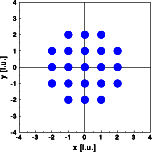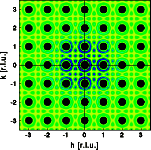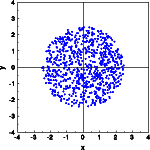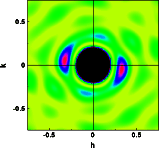Interactive Tutorial about Diffraction Convolution IV: Circular boxConvolution Convolution theorem Square box Rectangular box Circular box Box at 45 degrees Goto Contents In order to illustrate the theorem further, lets limit the crystal by a circular box (left image). Again the reciprocal lattice results convoluted by the Fourier transform of a rectangular box (right image). Click on the pictures to get a full screen version.As expected, the Bragg reflections are convoluted by the Fourier transform of the shape function again. This time there are circular maxima around the Bragg reflections. These features represent the Fourier transform of the shape function. Since the circular box has a small diameter, the resulting crystal is limited by a set of {100} and {110} faces, rather than by a circle. The form is, however, fair enough an approximation to a circle. The circular box is simulated by filling the shape at random with a large number (800) of atoms (left image). The resulting electron density distribution fills the box fairly equally. Next the Fourier transform is calculated in a small section of reciprocal space near the origin. The calculated intensity distribution corresponds to the distribution of the subsidiary maxima observed in the Fourier transform above.The deviations from circular symmetry are due to the limited statistics provided by the 800 atoms within the square box. © Th. Proffen and R.B. Neder, 2003